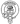# Introduction to CommonJS Modules

June 29, 2020

Despite the ECMAScript Module system being the official standard for modules in JavaScript, Node.js continues to use CommonJS by default. You can use the ES Module System in your Node projects by compiling your js with something like Babel but it’s worth learning the CJS syntax as well.

Before we deep-dive the syntax we first need to define what a module is in JavaScript:

A module is a JavaScript file that exports one or more values (objects, functions or variables), using the export keyword.

In order to import one of these modules we use `require`. Let’s take a look at a simple example:

``const package = require('module-name')``

And to export something from a module we add it to the exports like so:

``````// add.js

exports.add = (x, y) => x + y``````

This is just a simple function that takes two numbers, adds them together and returns the result.

Here’s how we would import and use our shiny new add module:

``````const addModule = require('./add.js')

// This add module can actually also be used to concatenate strings

You can also export more than one value like this:

``````// math.js

exports.add = (x, y) => x + y
exports.subtract = (x, y) => x - y
exports.multiply = (x, y) => x * y
exports.divide = (x, y) => x / y``````

Then we can import these exports individually by leveraging the destructuring syntax.

``````const { add, subtract, multiply, divide } = require('./math.js')

subtract(2, 1) // returns 1
multiply(3, 3) // returns 9
divide(4, 2) // returns 2``````

It’s even possible to export just a single value for a module, similar to how default exports work in ES Modules.

``````// math.js

const add = (x, y) => x + y

``````const add = require('./math.js')